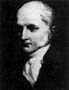Joseph Proust (1754 - 1826)Current Atomic Model1. He first published his Law of Definite Proportions (or Law of Constant Composition) in 1794.
2. This law states that a compound is composed of exact proportions of elements by mass regardless of how the compound was created. For example, to make water there is a specific ratio of grams of hydrogen to grams of oxygen regardless of where your sample of water was found. All water has this particular proportion.
3. For example, if one sample of water breaks down into 2 grams of hydrogen and 16 grams of water, then twice the amount of water would contain 4 grams of hydrogen and 32 grams of water. The proportion of hydrogen to oxygen is "definite" or fixed.